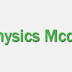01. One kilowatt-hour is the amount of energy delivered during _________?
A.one second
B.one minute
C.one day
D.one hour

02. How much heat does a 40 W bulb generates in one hour?
A.155000 j
B.144000 j
C.133000 j
D.122000 j

03.Resistance of a superconductor is ________?
A.finite
B.infinite
C.zero
D.Changes with every conductor

04. Which one is the best material for making connecting wires?
A.iron
B.copper
C.silver
D.tungsten

05. Internal resistance is the resistance offered by _______?
A.source of EMF
B.resistor
C.conductor
D. capacitor

06. The SI unit of heat is _______?
A.Watt
B.Joule
C.Volt
D.Newton

07. An electric iron marked 20 volts 500 W.The units consumed by it in using if for 24 hours will be _________?
A.10
B.12
C.14
D.16

08.The graphical representation of Ohms law is ________?
A.hyperbola
B.parabola
C.ellipse
D.straight line

09.Electrical energy is measured in _________?
A.Kilowatt-hour
B.kilo watt
C.horse power
D.watt

Physics Mcqs for Test Preparation from basic to AdvanceReviewed by Nokriads.com on April 17, 2020 Rating: 5